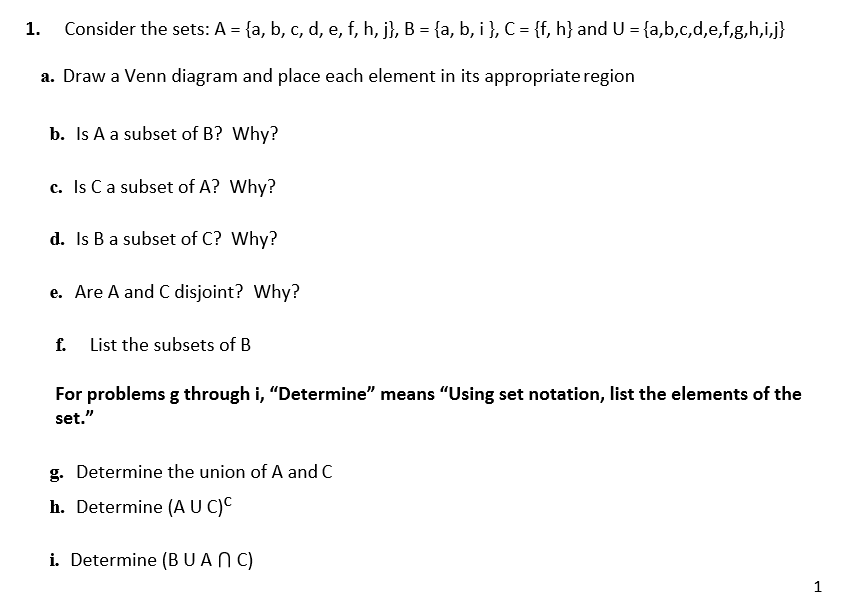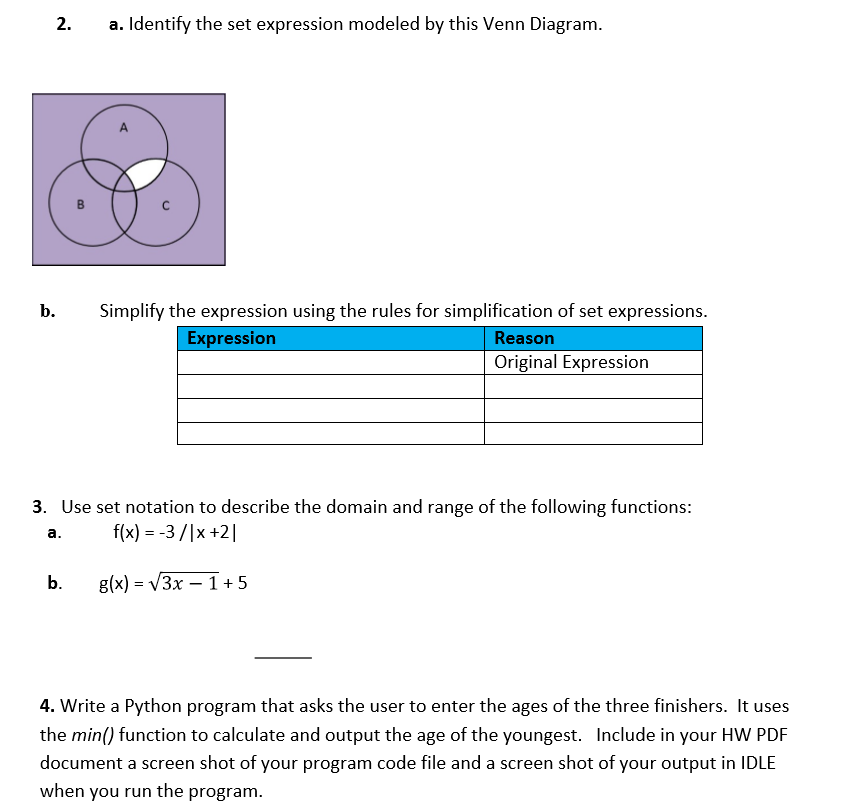# Consider the sets: A = {a, b, c, d, e, f, h, j}, B = {a, b, i}, C = {f, h} and U = {a,b,c,d,e,f,g,h,i,j}1.a. Draw a Venn diagram and place each element in its appropriate regionb. Is A a subset of B? Why?c. Is Ca subset of A? Why?d. Is B a subset of C? Why?e. Are A and C disjoint? Why?List the subsets of Bf.For problems g through i, "Determine" means "Using set notation, list the elements of theset."g. Determine the union of A and Ch. Determine (AU C)Ci. Determine (BUAN C) a. Identify the set expression modeled by this Venn Diagram.2.ASimplify the expression using the rules for simplification of set expressions.b.ExpressionReasonOriginal Expression3. Use set notation to describe the domain and range of the following functions:f(x) = -3 /|x +2|a.g(x) = V3x – 1+5b.4. Write a Python program that asks the user to enter the ages of the three finishers. It usesthe min() function to calculate and output the age of the youngest. Include in your HW PDFdocument a screen shot of your program code file and a screen shot of your output in IDLEwhen you run the program.

Question
28 viewshelp_outlineImage TranscriptioncloseConsider the sets: A = {a, b, c, d, e, f, h, j}, B = {a, b, i}, C = {f, h} and U = {a,b,c,d,e,f,g,h,i,j} 1. a. Draw a Venn diagram and place each element in its appropriate region b. Is A a subset of B? Why? c. Is Ca subset of A? Why? d. Is B a subset of C? Why? e. Are A and C disjoint? Why? List the subsets of B f. For problems g through i, "Determine" means "Using set notation, list the elements of the set." g. Determine the union of A and C h. Determine (AU C)C i. Determine (BUAN C) fullscreenhelp_outlineImage Transcriptionclosea. Identify the set expression modeled by this Venn Diagram. 2. A Simplify the expression using the rules for simplification of set expressions. b. Expression Reason Original Expression 3. Use set notation to describe the domain and range of the following functions: f(x) = -3 /|x +2| a. g(x) = V3x – 1+5 b. 4. Write a Python program that asks the user to enter the ages of the three finishers. It uses the min() function to calculate and output the age of the youngest. Include in your HW PDF document a screen shot of your program code file and a screen shot of your output in IDLE when you run the program. fullscreen
check_circle

Step 1

Hey, since there are multiple questions posted, we will answer first question (1) with first three subparts. If you want any specific question to be answered then please submit that question only or specify the question number with subparts in your me...

### Want to see the full answer?

See Solution

#### Want to see this answer and more?

Solutions are written by subject experts who are available 24/7. Questions are typically answered within 1 hour.*

See Solution
*Response times may vary by subject and question.
Tagged in

### Math